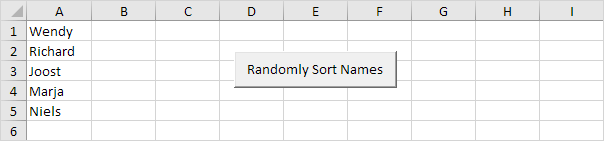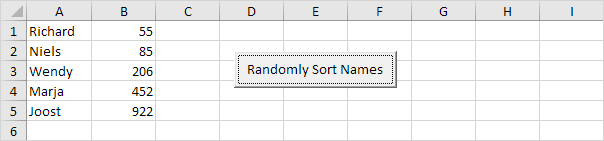# Randomly Sort Data

Below we will look at a program in Excel VBA that randomly sorts data (in this example randomly sorts names).

Situation:1. First, we declare four variables. One variable of type String we call TempString, one variable of type Integer we call TempInteger, one variable of type Integer we call i, and one variable of type Integer we call j.

Dim tempString As String, tempInteger As Integer, i As Integer, j As Integer

2. We write 5 random numbers to column B (one for each name). We use the worksheet function RandBetween for this.

For i = 1 To 5
Cells(i, 2).Value = WorksheetFunction.RandBetween(0, 1000)
Next i

Result so far:We will use the numbers next to each name to sort the names. The name with the lowest number first, the name with the second lowest number, second, etc.

3. We start a Double Loop.

For i = 1 To 5
For j = i + 1 To 5

4. Add the following code line:

If Cells(j, 2).Value < Cells(i, 2).Value Then

Example: for i = 1 and j = 2, Wendy and Richard are compared. Because Richard has a lower number, we swap Wendy and Richard. Richard is at the first position now. For i = 1 and j = 3, Richard and Joost are compared. Joost has a higher number so nothing happens. This way, Excel VBA gets the name with the lowest number at the first position. For i = 2, Excel VBA gets the name with the second lowest number at the second position, etc.

5. If true, we swap the names.

tempString = Cells(i, 1).Value
Cells(i, 1).Value = Cells(j, 1).Value
Cells(j, 1).Value = tempString

6. And we swap the numbers.

tempInteger = Cells(i, 2).Value
Cells(i, 2).Value = Cells(j, 2).Value
Cells(j, 2).Value = tempInteger

7. Don't forget to close the If statement.

End If

8. Don't forget to close the two loops.

Next j
Next i

9. Test the program.

Result:Note: you can add a line which deletes the numbers in column B. It's even nicer to place the numbers of each name in an array, so no numbers are placed on your worksheet. However, for illustrative purpose we've chosen to place the values on the sheet.

Go to Next Chapter: Macro Errors